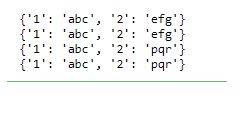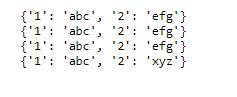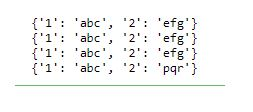/  Technology   /  Ways to copy a dictionary and edit the copy in Python## Ways to copy a dictionary and edit the copy in Python

Python do not implicitly copy the dictionary or any objects. When we set dict2 = dict1, it refers to the same dictionary object. Henceforth, even when we mutate the dictionary, all that are referred to it, keep referring to the object in its current state.

#### Example:

```#copy a dictionary and only edit the copy in Python
dict1 = {"1": "abc", "2": "efg"}

dict2 = dict1

print(dict1)
print(dict2)

dict2['2'] = 'pqr'

print(dict1)
print(dict2)```

Output:To copy a dictionary, we can either use a shallow copy or deep copy method, as explained in the below example.

### Using shallow copy

#### Example:

```#Using shallow copy
dict1 = {"1": "abc", "2": "efg"}

print(dict1)

dict3 = dict1.copy()
print(dict3)

dict3['2'] = 'xyz'

print(dict1)
print(dict3)```

Output:### Using deep copy

#### Example:

```#Using deep copy
import copy

dict1 = {"1": "abc", "2": "efg"}

print(dict1)

dict4 = copy.deepcopy(dict1)
print(dict4)

dict4['2'] = 'pqr'

print(dict1)
print(dict4)```

Output: